# Thermal Performance of EAHE System in Algerian Site: Case Study of Ghardaïa

Thermal Performance of EAHE System in Algerian Site: Case Study of Ghardaïa

Khalfallah Benbelgacem Abdelmadjid Kaddour Salah Bezari Younes Menni Chutarat TearnbuchaGiulio Lorenzini Weerawat Sudsutad Hijaz Ahmad

Department of Physics, LENREZA Laboratory, UKM Ouargla University, Ouargla 30000, Algeria

Unité de Recherche Appliquée en Energies Renouvelables, URAER, Centre de Développement des Energies Renouvelables, CDER, Ghardaïa 47133, Algeria

Department of Technology, University Centre of Naama (Ctr Univ Naama), P.O. Box 66, Naama 45000, Algeria

Department of General Education, Faculty of Science and Health Technology, Navamindradhiraj University, Bangkok 10300, Thailand

Department of Engineering and Architecture, University of Parma, Parco Area delle Scienze, 181/A, Parma 43124, Italy

Department of Statistics, Faculty of Science, Ramkhamhaeng University, Bangkok 10240, Thailand

Section of Mathematics, International Telematic University Uninettuno, Corso Vittorio Emanuele II, 39, Roma 00186, Italy

Corresponding Author Email:
chutaratt@nmu.ac.th
Page:
375-382
|
DOI:
https://doi.org/10.18280/ijdne.170307
1 April 2022
|
Accepted:
6 June 2022
|
Published:
30 June 2022
| Citation

OPEN ACCESS

Abstract:

Among the renewable energies there is geothermal energy, conventional air conditioning has negative consequences on the environment, to have an economical and sustainable solution: the EAHE respond to this concern. In the current study, the study of the thermal performance of the earth-air heat exchanger (EAHE) has been completed. For the impact of transient heat transfer in the ground near the hose, the thermal saturation of the ground is well mentioned. In addition, the role of the burial depth of the EAHE system is also taken into account during this work. The suitable depth of the buried tubes was determined by implementing the thermo-physical properties of the soil of the studied site located in Ghardaïa (Algeria) by writing a specific program. A parametric investigation was accomplished the effects of the length and the diameter of the pipe, in addition the speed of the inlet air on the temperature of the outlet of the EAHE system in both cases PVC and HDPE material. The results showed that the optimal parameters in order to obtain a good performance for the studied EAHE: maximal value of pipe length, and minimal values of diameter pipe and air velocity 30 mm and 1.2 m/s respectively.

Keywords:

earth-air heat exchanger, parametric analysis, soil, temperature, PVC, HDPE, passive cooling

1. Introduction

The useful exploitation of renewable energies such as solar sources can generate remarkable effects such as the consumption of fossil fuels, energy cost. The cooling and the heating of the building are solutions for the energy optimization of the building [1, 2]. Reducing the heating and cooling energy consumption of the building via such energy with no CO2 emission as geothermal energy, as an application of the EAHE system [3, 4].

In current research, much of the work has been directed towards the use of renewable energies in various fields such as photovoltaic PV , the buildings [6, 7], and agricultural greenhouses . As an application of geothermal energy, air-soil exchangers; they are considered one of the sustainable and economic solutions. Dynamic thermal simulation of an EAHE heat exchanger integrated into a building is located in Morocco, using the TRNSYS software was implemented .

Many researchers in their work have focused on improving cooling systems, including assessments of their economic impact. D’Agostino et al.  revealed that two thermal performance factors for EAHE resulting in good heat transfer: firstly, smaller diameters of the tubes, and secondly a length between 80 and 100 m. Al-Helal et al.  showed that the EAHE exchanger works in an interesting way at 3 m depth, resulting a remarkable cooling/heating load. The cooling and heating of the EAHE in both winter and summer seasons via a theoretical calculation was analyzed . The different studies in several impacts of an earth-air heat exchanger in semi-arid and arid regions were reviewed . The decrease in the outlet temperature of the EAHE system is caused by high flow velocities . Khabbaz et al.  validated their model by the experimental study via the TRNSYS software, in addition, they found that the temperature inside the exchanger stabilizes at a hose length of 72 m and a depth of 3.2 m. The best duality is (D = 0.2 m and V = 3 m/s) in order to generate the optimal cooling load with a payback period of 6.35 years .

An EAHE system evaluation including its thermal performance which allows thermal comfort by renewable and clean means . The comparison of the EAHE system with the split AC system is well done via a soil potential study . The sandy soil is the best ground cover material to improve the performance of EAHE system . The mass flow rate increase behavior is similar to that of the cooling load required in the EAHE system .

Basok et al.  validated a numerical model of the circular EAHE system. A longer pipe with a smaller buried diameter having a lower airflow velocity generates an optimal performance of the EAHE system . Michalak et al.  showed that a study of the operation of a building connected to EAHE model via simple thermal dynamics.

The main objective of this work is to study the EAHE system performance under Ghardaïa (Algeria) climate conditions. A parametric analysis was completed to know the impact of geometric, dynamic parameters on the EAHE outlet temperature.

2. Climatic Data Case Study

The climate of the Ghardaïa region (32.36° North, 3.51° West) is characterized by hot and cold seasons summer and winter respectively. A variable very low amount of precipitation in the year. This work has been exploited the data measured within the URAER unit (Ghardaïa, Algeria) and this using a meteorological station (Figure 1).

## 1.png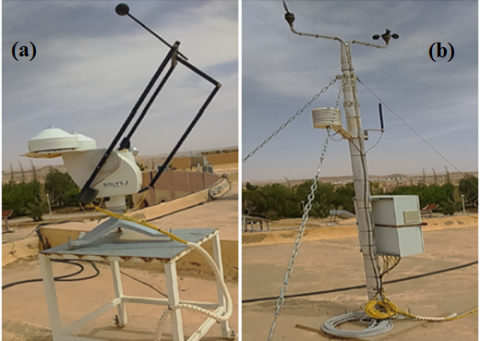Figure 1. (a) Radiometric station, (b) meteorological station at URAER-Ghardaïa

Figure 2 indicates the evolution per month of the annual global solar radiation during the year 2017, the months of winter (December, January, February) represent a minimum of global solar radiation which reaches the value 3.4 kWh/m2, unlike during the summer period this value can reach 7.8 kWh/m2 in July.

## 2.png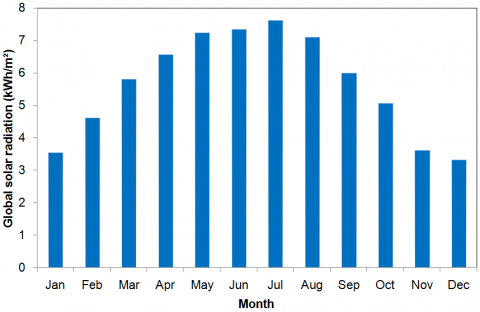Figure 2. Monthly variation of the global solar radiation in Ghardaïa

## 3.png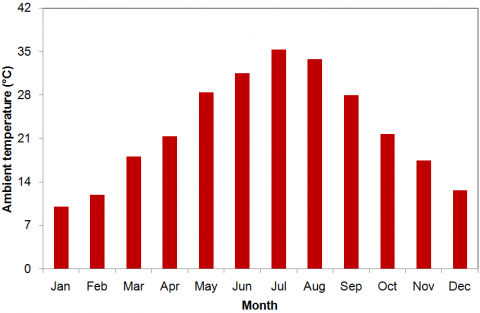Figure 3. Monthly variation of the ambient temperature in Ghardaïa

According to Figure 3, the average annual temperature in Ghardaïa is 22.4℃, of which the hottest temperature recorded in the month of July reaches the value of 36℃, on the other hand, the lowest temperature measured in January is 9.8℃.

The mean amount of relative humidity for the year in Ghardaïa is 39.4%. Both measured values of RH of 20.3% and 56.8% in the months of July and January respectively (Figure 4).

## 4.png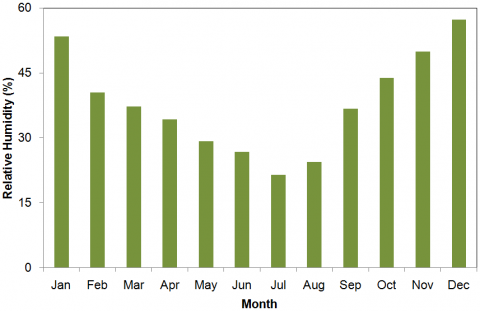Figure 4. Monthly variation of the relative humidity in Ghardaïa

3. System Description

EAHE systems are utilized to optimize the heating and cooling needs of buildings. The heat exchanger is a tube, usually made of PVC and HDPE, that is buried underground. The principle of operation of the EAHE system is as follows: The outside air is blown with a continuous supply into the buried pipe, and its temperature becomes very close to the temperature of the earth due to the heat exchange taking place between it and the earth, and then it is pumped into space as shown in Figure 5.

To analyze the effect for different design, operation factors on the EAHE efficiency, the thermo-physical characteristics of air, pipe materials are considered in Table 1.

## 5.png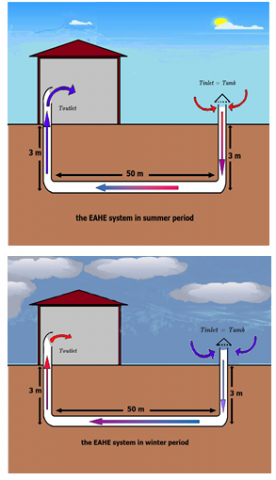Figure 5. Explanatory diagram of EAHE system

Table 1. Two EAHE system properties

 Material Density (kg/m3) Thermal conductivity (W/m.K) Specific heat (J/kg.K) Air  1.1774 0.02624 1005.7 PVC  1400 0.17 900 HDPE  950 0.45-0.52 1900

Table 2 gives the thermo-physical properties of the soil. The parameters used in our parametric analysis of EAHE systems are shown in Table 3.

Table 2. Thermo-physical properties of soil 

 Characteristics Values Thermal conductivity 5.1 (W/m.K) Specific heat 652 (J.kg/℃) Density 2600 (Kg/m3) Average annual temperature 21.7 (℃)

Table 3. Parameters used in the analysis

 Parameters Values Pipe length (L) 60 to 100 m Internal diameter (Di) 30 mm - 90 mm Pipe thickness 4 mm Air velocity (V) 1.2 to 3.2 m/s Pipe depth 3 m
4. Mathematical Modelling

4.1 Soil temperature modeling

The soil is a limited semi-infinite medium on its upper surface by a plane on which a periodic temperature signal is applied. Soil temperature analysis will be done using a mathematical model of periodic conduction based on Fourier’s equation:

$\frac{\partial^{2} T}{\partial z^{2}}-\frac{1}{\alpha} \frac{\partial T}{\partial t}=0$                        (1)

$T(0, t)=T_{\text {mean }}+A_{a} \cos \left(\omega\left(t-t_{0}\right)\right)$                        (2)

$T(\infty, t)=T_{\text {mean }}$                  (3)

where the soil thermal diffusivity was expressed as the following form:

$\alpha=\frac{\lambda}{\rho C_{p}}$                       (4)

The soil temperature is given by:

$T(z, t)=T_{\text {mean }}+A_{s}\left(\operatorname{Exp}-(z) \sqrt{\frac{\pi}{365 \alpha}} \cos \left\{\frac{2 \pi}{365}\left(t-t_{0}\right)-(z / 2) \sqrt{\frac{\pi}{365 \alpha}}\right\}\right)$                  (5)

At the outlet of EAHE, the air temperature is predicted as follows :

$T_{s}=T_{a m b}+\left(T(z, t)-T_{a m b}\right) \times\left(1-e^{-\frac{G_{T o t}}{\dot{m} C_{P f}} x}\right)$                        (6)

4.2 Modeling of EAHE

The modeling of the EAHE system is based on models developed in literature [27, 28]. The coefficient of heat transfer by convection in a tube is defined by:

$h_{c o n v}=\frac{N u \lambda}{D}$                    (7)

The number of Nusselt was expressed by the following correlation:

$N u=0.0214\left(R e^{0.8}-100\right) P r^{0.4}$                  (8)

Re and Pr are given by:

$R e=\frac{V_{\operatorname{air}} D_{i}}{v}$                  (9)

$P r=\frac{v \rho c p}{\lambda}$                    (10)

The heat transfer is given by:

$\phi=\dot{m} C_{P f} d T(x)$                    (11)

$\phi=\frac{d x}{R_{\text {conv }}+R_{\text {pipe }}+R_{\text {soil }}}(T(z, t)-T(x))$                   (12)

The thermal resistance of the hose:

$R_{\text {pipe }}=\frac{1}{\lambda_{\text {pipe }} 2 \pi} \ln \left(r_{e} / r_{i}\right)$                   (13)

The convective thermal resistance is:

$R_{\text {conv }}=\frac{1}{r_{i} h_{\text {conv }} 2 \pi}$                  (14)

The thermal resistance of the soil is determined by:

$R_{\text {soil }}=\frac{1}{\lambda 2 \pi} \ln \left(R_{(z, t)} / r_{e}\right)$                     (15)

Then, the total heat transfer coefficient of the EAHE:

$G_{\text {Tot }}=\frac{1}{\left(R_{\text {conv }}+R_{\text {pipe }}+R_{\text {soil }}\right)}$                     (16)

After the combination of the two Eqns. (12) and (16), the energy balance is as follows:

$\frac{d T(x)}{T(z, t)-T(x)}=\frac{G_{T o t}}{\dot{m} C_{P f}} d x$                       (17)

Integrating Eq. (17) is then:

$-\ln (T(z, t)-T(x))=\frac{G_{T o t}}{\dot{m} C_{P f}} x+C t e$                       (18)

The boundary equation at the ground surface is:

$T(0)=T_{a m b}$                   (19)

The following equation is obtained when the constant in Eq. (18) is replaced by its expression deduced from Eq. (19):

$\ln \left(T(x)-T(z, t)\right) /\left(T_{a m b}-T(z, t)\right)=\frac{-G_{T o t}}{\dot{m} C_{P f}} x$                   (20)

Finally, the air temperature can be calculated at the outlet (x = L) as follows:

$T_{s}=T_{a m b}+\left(T(z, t)-T_{a m b}\right)\left(1-e^{-\frac{G_{T o t}}{\dot{m} C_{P f}} x}\right)$                    (21)

The simulation is based on the discretization of EAHE system with a length of 50 m and divided in 25 parts each of 2 meters. The equations given above are solved for each part of the EAHE with a program written in Scilab software (Figure 6).

According to the experimental measurements, the annual temperature fluctuation of the underground soil below a certain depth of 5 m stabilizes throughout the year (Figure 7). In order to obtain a temperature distribution inside the buried hose, the constant temperature boundary condition for the ground surface. In the present study, both boundary conditions can be used.

## 6.png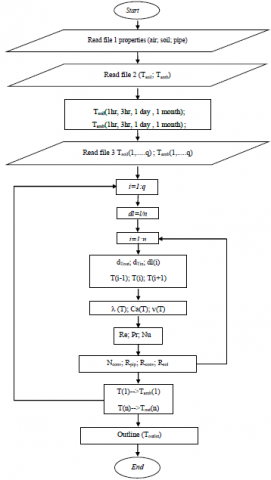Figure 6. Flowchart of numerical model

## 7.png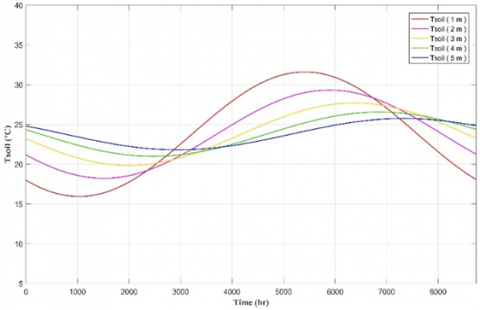Figure 7. The annual ground temperature for several depths

5. Results and Discussions

5.1 Effect of pipe’s diameter

A reduction in convective heat transfer coefficient results for higher values of inside diameter of the pipe generates high and low temperatures in summer, in winter respectively. (Figures 8 and 9), the optimal diameter for our study is 30 mm, it corresponds to difference of outlet temperature of 11.6222℃.

## 8.png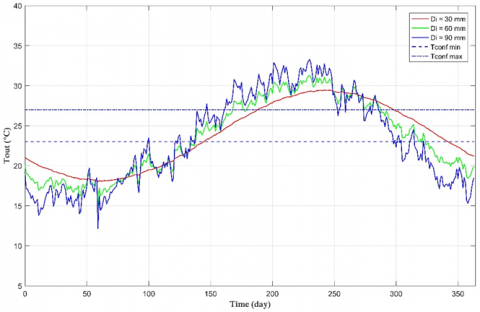Figure 8. Effect of the pipe inner diameter on Tout (PVC)

## 9.png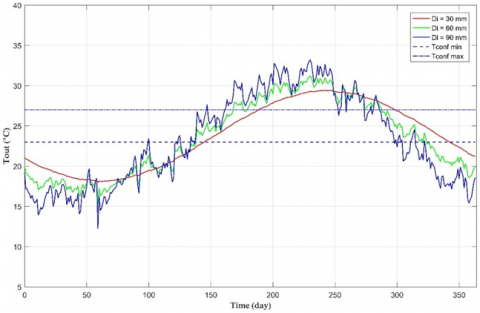Figure 9. Effect of the pipe inner diameter on Tout (HDPE)

Table 4 shows the difference $\Delta$Tout of EAHE system in both cases (PVC and HDPE). There is no great influence between the two materials on this temperature difference.

Table 4. Variation of difference of outlet temperature with respect to pipe diameter of EAHE

 Di (mm) ΔTout(℃) (PVC) ΔTout(℃) (HDPE ) 30 11.6222 11,5361 60 17.9304 17,6185 90 24.9555 24,657

With: $\Delta$ Tout $_{\text {Di }}=\mid$ Tout $_{\text {Dimax }}-$ Tout $_{\text {Dimin }} \mid .$

5.2 Effect of velocity of the air

According to Figures 10 and 11, the outlet temperature of the EAHE system is dependent on the air velocity profile, for velocity values of 1.2 m/s the temperature Everything is close to the thermal comfort zone (23 - 27)℃, contrary to the values of 3.2 m/s.

The data in Table 5 shows the difference of EAHE outlet temperature for several air velocities. This parameter ΔTout increase for higher velocities. In the case: HDPE pipe, the value of this mentioned parameter is less than the case of PVC pipe.

## 10.png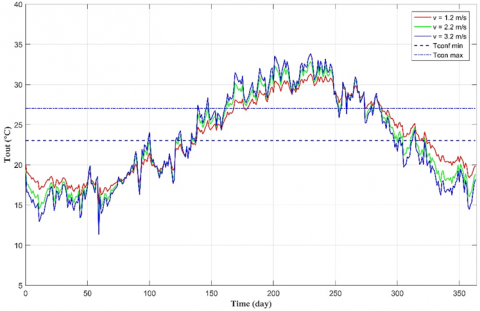Figure 10. Influence of the air velocity on the annual air outlet temperature (PVC)

## 11.png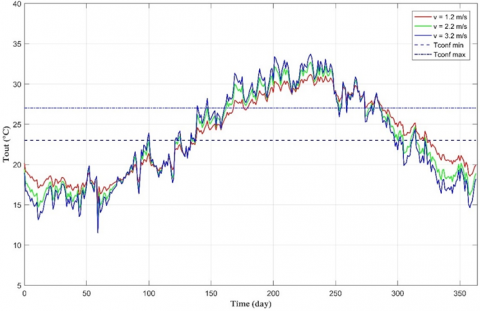Figure 11. Influence of the air velocity on the annual air outlet temperature (HDPE)

Table 5. Air velocity influence on difference of EAHE exist temperature

 Vi (m/s) ΔTout(℃) (PVC) ΔTout(℃) (HDPE ) 1.2 16.9304 11.5361 2.2 23.2463 17.6185 3.2 26.9646 26.657

With: $\Delta$ Tout $_{V i}=\mid$ Tout $_{\text {Vimax }}-$ Tout $_{\text {Vimin }} \mid .$

5.3 Effect of pipe length

According to Figures 12 and 13, we observe a decrease in the temperature of the air at the outlet of the EAHE exchanger with the increase in the length of the pipe. An optimum annual air outlet temperature corresponds to a pipe length of 100 m (near the area of thermal comfort (23-27)℃), On the contrary, this annual average temperature of the outlet has a value a little far from the thermal comfort zone.

When the pipe length increases, the exchange surface increases also and since the quantity of heat lost by air is proportional to the exchange surface, therefore the air temperature will decrease further.

Table 6 shows the variation in difference of EAHE outlet temperature for three values of pipe lengths. The optimal functioning of EAHE corresponds to the minimum values of ΔTout with L = 100 m.

## 12.png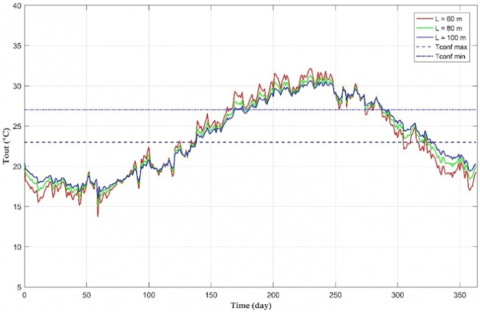Figure 12. Influence of the length of hose on the annual air outlet temperature (PVC)

## 13.png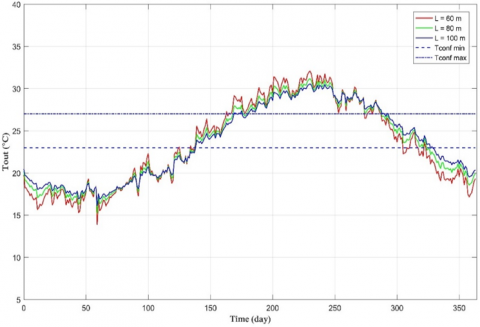Figure 13. Influence of the length of hose on the annual air outlet temperature (HDPE)

Table 6. Variation of difference of EAHE outlet temperature   with respect to pipe length

 Li (m) ΔTout(℃) (PVC) ΔTout(℃) (HDPE ) 60 20.9070 20.5581 80 17.9304 17.6185 100 15.8855 15.6210

With $\Delta$ Tout $_{\mathrm{Li}}=\mid$ Tout $_{\mathrm{Limax}}-$ Tout $_{\mathrm{Limin}} \mid$.

6. Conclusions

In this present paper, we studied an earth-to-air heat exchanger intended to the cooling of buildings under the climatic conditions of the Sahara site (Ghardaïa region, Algeria). This heat exchanger is constituted of two materials type PVC and HDPE buried at a depth of 3 m in soil. We chose like period of study for full year. After we have simulated the system via the Scilab software, we led to the following results:

• The increase in air temperature is essential during the penetration of air into the high temperature pipe.
• The air outlet temperature is as much weaker than the heat exchanger is longer whereas it increases with the growing of the section of the tube and the speed of air. The influence of the hose thickness on the EAHE outlet temperature is negligible.
• The HDPE material is little better than PVC by 1% more.
• Tube diameter, tube length, air velocity, burial depth and soil nature are the most impactful parameters.
• A good performance for the studied EAHE: with maximal value of pipe length around 100 m.
Acknowledgment

Chutarat Tearnbucha would like to acknowledge financial support by Navamindradhiraj University through the Navamindradhiraj University Research Fund (NURF).

Nomenclature
 D pipe diameter, m Cp specific heat, J. kg-1. K-1 x elementary part of pipe length, m L pipe length, m k thermal conductivity, W.m-1. K-1 z depth from the earth surface, m V air velocity, m/s t time, hour Re Reynolds number Nu Nusselt number Pr Prandtl number T temperature, ℃ Ts air outlet temperature of EAHE ℃ hconv coefficient of heat transfer by convection in a tube, W.m-2.K-1 Rpipe thermal resistance of the pipe, m.K.W-1 Rconv convective thermal resistance, m.K.W-1 Rsoil thermal resistance of the soil, m.K.W-1 GTot total thermal conductance of the EAHE, W.m-1.K-1 Greek symbols $\alpha$ thermal diffusivity, m2. s-1 $\beta$ thermal expansion coefficient, K-1 $\phi$ solid volume fraction Ɵ dimension less temperature µ dynamic viscosity, kg. m-1.s-1 Subscripts EAHE earth–air heat exchanger PVC polyvinyl chloride HDPE High Density Poly Ethylene
References

 Zarezade, M., Mostafaeipour, A. (2016). Identifying the effective factors on implementing the solar dryers for Yazd province, Iran. Renewable and Sustainable Energy Reviews, 57: 765-775. https://doi.org/10.1016/j.rser.2015.12.060

 Rabani, M., Bayera Madessa, H., Mohseni, O., Nord, N. (2020). Minimizing delivered energy and life cycle cost using Graphical script: An office building retrofitting case. Applied Energy, 268: 114929. https://doi.org/10.1016/j.apenergy.2020.114929

 Mostafaeipour, A., Goudarzi, H., Khanmohammadi, M., Jahangiri, M., Sedaghat, A., Norouzianpour, H., Chowdhury, S., Techato, K., Issakhov, A., Almutairi, K., Hosseini Dehshiri, S.J. (2021). Techno-economic analysis and energy performance of a geothermal earth-to-air heat exchanger (EAHE) system in residential buildings: A case study. Energy Science & Engineering, 9(10): 1807-1825. https://doi.org/10.1002/ese3.952

 Sakhri, N., Menni, Y., Ameur, H. (2020). Experimental investigation of the performance of earth-to-air heat exchangers in arid environments. Journal of Arid Environments, 180: 104215. https://doi.org/10.1016/j.jaridenv.2020.104215

 Kaddour, A., El, S.M., Bekkouche, A., Bezari, S., Benyoucef, B. (2018). Optimization and evaluation of the photovoltaic system in a farm studio located in Ghardaïa. In 6th International Renewable and Sustainable Energy Conference (IRSEC), pp. 1-4. https://doi.org/10.1109/IRSEC.2018.8702279

 Kaddour, A., Bekkouche, S.M.A. (2018). Natural ventilation around and through building: A numerical study. In Exergy for A Better Environment and Improved Sustainability 2, pp. 533-546. https://doi.org/10.1007/978-3-319-62575-1_38

 Kaddour, A., Bezari, S., Tearnbucha, C., Sudsutad, W., Lorenzini, G., Ahmad, H., Menni, Y. (2022). Simulation investigation of natural ventilation on the thermal comfort in arid regions: Case Ghardaïa. International Journal of Design & Nature and Ecodynamics, 17(1): 9-15. https://doi.org/10.18280/ijdne.170102

 Bezari, S., Amine Bekkouche, S.M.E., Benchatti, A., Adda, A., Boutelhig, A. (2020). Effects of the rock-bed heat storage system on the solar greenhouse microclimate. Instrumentation Mesures Métrologies, 9(6): 471-479. https://doi.org/10.18280/i2m.190608

 Arroub, I., Bahlaoui, A., Belhouideg, S., Kaddour, A. (2021). Dynamic modelling of an earth-to-air heat exchanger for air cooling on the building in hot temperate climate of Beni Mellal Morocco. International Journal of Energetica (IJECA), 13: 4.

 D’Agostino, D., Esposito, F., Greco, A., Masselli, C., Minichiello, F. (2020). Parametric analysis on an earth-to-air heat exchanger employed in an air conditioning system. Energies, 13(11): 2925. https://doi.org/10.3390/en13112925

 Al-Helal, I., Alsadon, A., Marey, S., Ibrahim, A., Shady, M., Abdel-Ghany, A. (2022). Geothermal energy potential for cooling/heating greenhouses in hot arid regions. Atmosphere, 13(1): 105. https://doi.org/10.3390/atmos13010105

 Cao, S., Li, F., Li, X., Yang, B. (2021). Feasibility analysis of earth-air heat exchanger (EAHE) in a sports and culture center in Tianjin, China. Case Studies in Thermal Engineering, 26: 101054. https://doi.org/10.1016/j.csite.2021.101054

 Sakhri, N., Menni, Y., Chamkha, A., Salmi, M., Ameur, H. (2020). Earth to air heat exchanger and Its applications in arid Regions - An updated review. TECNICA ITALIANA-Italian Journal of Engineering Science, 64(1): 83-90. https://doi.org/10.18280/ti-ijes.640113

 Jakhar, S., Misra, R., Soni, M.S., Gakkhar, N. (2016). Parametric simulation and experimental analysis of earth air heat exchanger with solar air heating duct. Engineering Science and Technology, an International Journal, 19(2): 1059-1066. https://doi.org/10.1016/j.jestch.2016.01.009

 Khabbaz, M., Benhamou, B., Limam, K., Hamdi, H., Hollmuller, P., Bennouna, A. (2015). Experimental and numerical study of an earth-to-air heat exchanger for buildings air refreshment in Marrakech. In 14th Conference of International Building Performance Simulation Association.

 Bhusare, H., Agrawal, K.K., Misra, R., Das, G. (2019). Techno-Economic analysis of earth air heat exchanger system for building cooling in hot and dry climate of Rajasthan (India). International Journal of Scientific & Engineering Research, 10(5): 23-28.

 Ahmed, A., Ip, K., Miller, A., Gidado, K. (2009). Thermal performance of earth-air heat exchanger for reducing cooling energy demand of office buildings in the United Kingdom. In 11th Conference of International Building Performance Simulation Association, pp. 2228-2235.

 Astina, I.M., Nugraha, M.Y. (2021). Numerical simulation of earth-air heat exchanger application for Indonesian simple house air conditioning system. Case Studies in Thermal Engineering, 28: 101371. https://doi.org/10.1016/j.csite.2021.101371

 Alkhalaf, H., Ibrahim, M.N., Yan, W. (2018). Numerical study about improving the proficiency of an earth air heat exchanger system (EAHE) employing ground cover material. Journal of Clean Energy Technologies, 6(2): 106-111. https://doi.org/10.18178/JOCET.2018.6.2.443

 Hasan, M.I., Muter, D.M. (2021). Numerical investigation of the air flow rate effect performance of earth to air heat exchanger used for cooling of poultry houses. Journal of Advanced Research in Fluid Mechanics and Thermal Sciences, 84(2): 167-184. https://doi.org/10.37934/arfmts.84.2.167184

 Basok, B., Bozhko, I., Novitska, M., Nedbailo, A., Tkachenko, M. (2021). Numerical modeling and experimental studies of the operational parameters of the earth-to-air heat exchanger of the geothermal ventilation system. Rocznik Ochrona Srodowiska, 23: 42-64. https://doi.org/10.54740/ros.2021.003

 Bisoniya, T.S. (2015). Design of earth–air heat exchanger system. Geothermal Energy, 3(1): 1-10. https://doi.org/10.1186/s40517-015-0036-2

 Michalak, P. (2022). Hourly simulation of an earth-to-air heat exchanger in a low-energy residential building. Energies, 15(5): 1898. https://doi.org/10.3390/en15051898

 Bansal, V., Misra, R., Agrawal, G., Mathur, J. (2009). Performance analysis of earth–pipe–air heat exchanger for winter heating. Energy and Buildings, 41(11): 1151-1154. https://doi.org/10.1016/j.enbuild.2009.05.010

 Bezari, S., Bekkouche, S.M., Benchatti, A. (2021). Investigation and improvement for a solar greenhouse using sensible heat storage material. FME Transactions, 49(1): 154-162.

 Kopeliovich, D. (2013). Thermoplastic High Density Polyethylene (HDPE). https://www.substech.com/dokuwiki/doku.php?id=thermoplastic_high_density_polyethylene_hdpe, accessed on 30 March 2022.

 Belatrache, D., Bentouba, S., Bourouis, M. (2017). Numerical analysis of earth air heat exchangers at operating conditions in arid climates. International Journal of Hydrogen Energy, 42(13): 8898-8904. https://doi.org/10.1016/j.ijhydene.2016.08.221

 Derbel, H.B.J., Kanoun, O. (2010). Investigation of the ground thermal potential in Tunisia focused towards heating and cooling applications. Applied Thermal Engineering, 30(10): 1091-1100. https://doi.org/10.1016/j.applthermaleng.2010.01.022